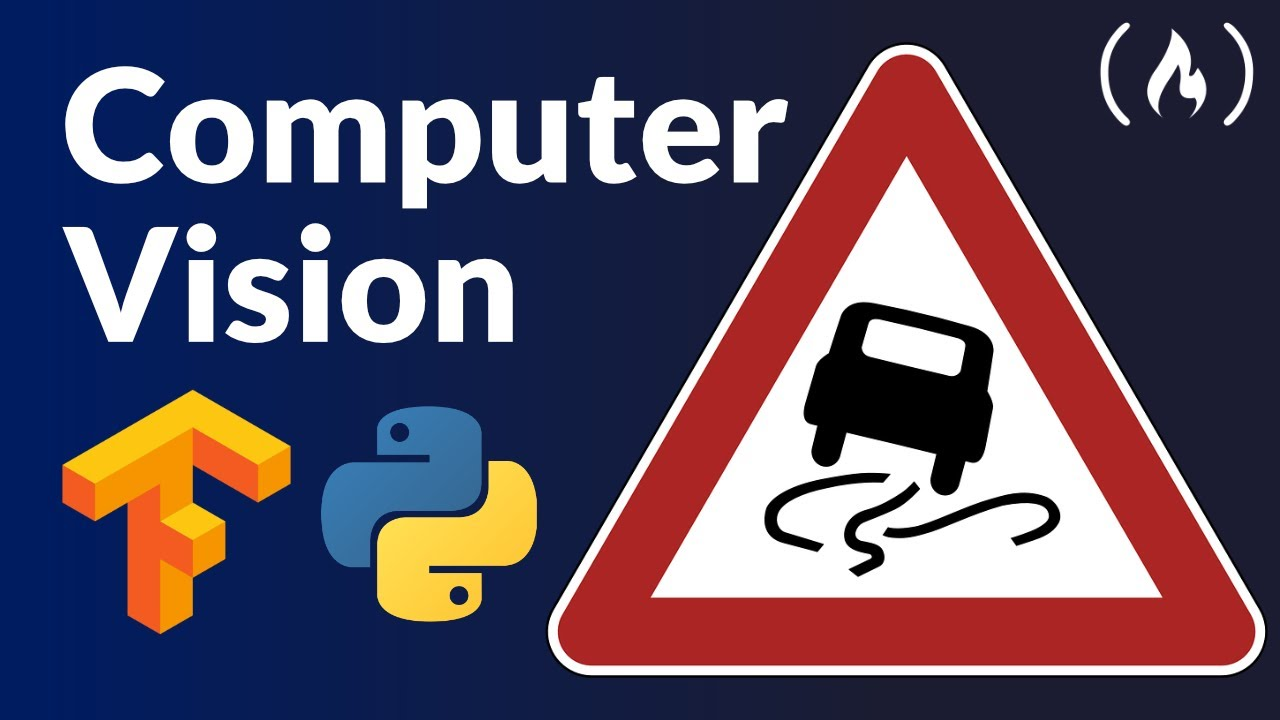# TensorFlow for Computer Vision Course - Full Python Tutorial for BeginnersLearn how to use TensorFlow 2 and Python for computer vision in this complete course. The course shows you how to create two computer vision projects.

Learn how to use TensorFlow 2 and Python for computer vision in this complete course. The course shows you how to create two computer vision projects. The first involves an image classification model with a prepared dataset. The second is a more real-world problem where you will have to clean and prepare a dataset before using it.

💻 Code: https://github.com/sniper0110/IntroductionToTensorflow2
✏️ Nour Islam Mokhtari created this course. Connect with him here: https://withkoji.com/@Nour_Islam

🔗 Get Nour’s free Machine Learning job-ready checklist: https://www.aifee.co/free-resources

⭐️ Course Contents ⭐️
⌨️ (0:00:00) Introduction
⌨️ (0:01:21) Course outline
⌨️ (0:05:11) Who’s this course for
⌨️ (0:05:35) Why learn TensorFlow
⌨️ (0:06:25) We will be using an IDE and not notebooks
⌨️ (0:10:50) Miniconda - how to install it
⌨️ (0:13:23) Miniconda - why we need it
⌨️ (0:17:24) How are we going to use conda virtual environments in VS Code?
⌨️ (0:21:20) Installing Tensorflow 2 (CPU version)
⌨️ (0:29:56) Installing Tensorflow 2 (GPU version)
⌨️ (0:43:34) What do we want to achieve?
⌨️ (0:45:26) Exploring MNIST dataset
⌨️ (1:05:54) Tensorflow layers
⌨️ (1:09:44) Building a neural network the sequential way
⌨️ (1:27:22) Compiling the model and fitting the data
⌨️ (2:00:52) Building a neural network the functional way
⌨️ (2:08:33) Building a neural network the Model Class way
⌨️ (2:14:31) Things we should add
⌨️ (2:18:29) Restructuring our code for better readability
⌨️ (2:23:11) First part summary
⌨️ (2:24:12) What we want to achieve
⌨️ (2:34:20) Preparing train and validation sets
⌨️ (2:53:37) Preparing the test set
⌨️ (3:10:17) Building a neural network the functional way
⌨️ (3:22:12) Creating data generators
⌨️ (3:31:39) Instantiating the generators
⌨️ (3:35:37) Compiling the model and fitting the data